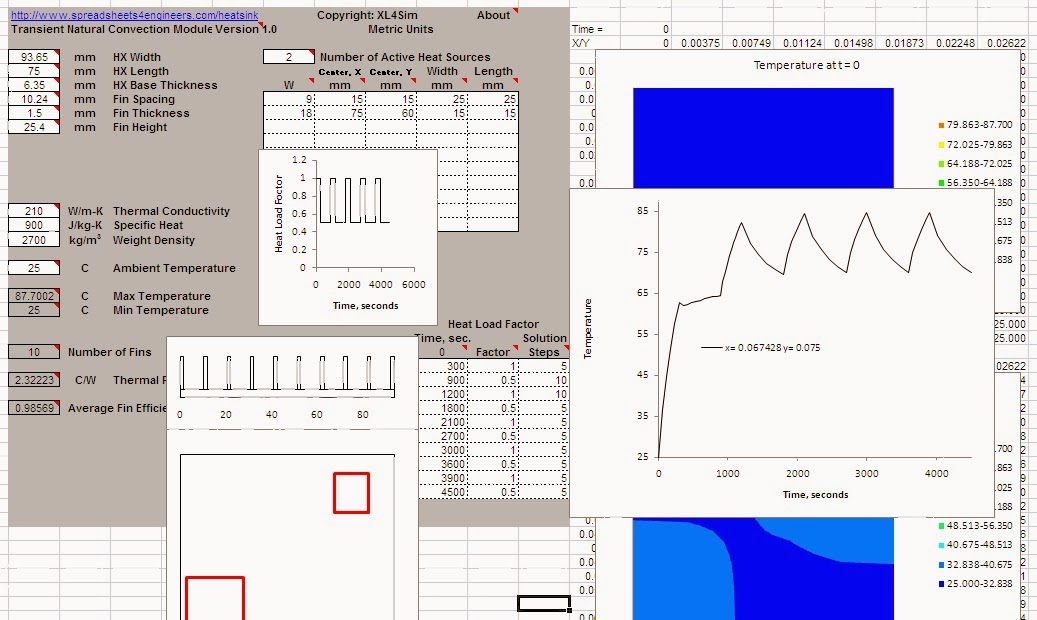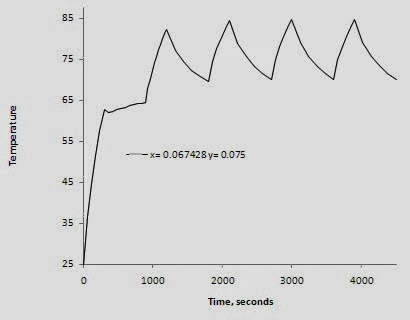## Tuesday, January 28, 2014

### Heat Sink Transient Response Benefits

An example of a natural convection finned heat sink is used to show how the thermal mass of the heat sink can be used to reduce its required cooling capacity.  Generally the effects of thermal mass are intuitive but calculating the benefits can sometimes be challenging.  This example shows an easy and quick simulation for evaluating the potential benefits of the transient behavior.XL4Sim Simulation of a Transient, Naturally Cooled Heat Sink.
A typical natural convection heat sink is used. It is 93.65 mm wide and 75 mm long with a base plate thickness of 6.35 mm, fin spacing of 10.14 mm, fin thickness of 1.5 mm and fin height of 24.5 mm - see the attached.  Two heat sources are used, 9 and 18 W located and sized as shown.  The heat load duty cycle is 5 minutes at full power followed by 10 minutes at 1/2 power.  The heat sink is assumed to be oriented with the fin channels vertical in free air at 25 C.

The maximum predicted heat sink temperature is 87.7 C for a corresponding delta T = 62.7 C.  The time/temperature plot is shown below for the maximum temperature location.Time/Temperature Plot.
After ~ 4 full power cycles the temperature has peaked.  This animation shows the heat sink temperatures over the heat cycles.Animation of the HX Temperatures over Time.
If the full power heat load is conservatively used then the steady state maximum predicted temperature is 98.9 C or a delta  T = 73.9 C.  This is a ~ 18% increase over the transient solution.Steady Temperatures Assuming Continuous Full Power.
If the cyclic heat load was predictably constant then the heat sink could conceivably be reduced in size for space and expense savings.

The above analysis was done using the Heat Sink module of the XL4Sim series.  The full analysis was setup and completed in less than 2 hours demonstrating the ease of use and efficiency of the simulation tool.